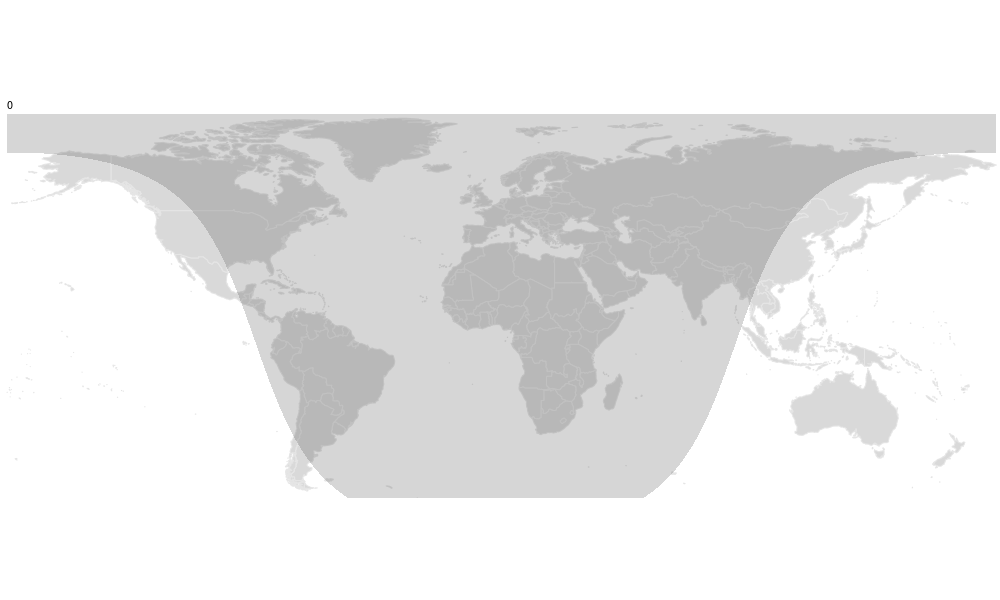# World map showing day and night regions

I'm trying to add a daytime/nighttime line to a world map using `ggplot` in order to indicate day and night regions; something like this:The plan is to animate my map over a 24 hour cycle like this:The above animation is achieved using a sine wave, which I know is totally inaccurate. I'm aware that `geosphere::gcIntermediate` allows me to draw great-circle lines, like so:

``````library(ggplot2)
library(ggthemes)
library(geosphere)

sunPath1 <- data.frame(gcIntermediate(c(-179, -30), c(0, 30), n=100))
sunPath2 <- data.frame(gcIntermediate(c(0, 30), c(179, -30), n=100))
sunPath <- rbind(sunPath1, sunPath2)

ggplot(sunPath) +
borders("world", colour = "gray95", fill = "gray90") +
geom_ribbon(aes(lon, ymax = lat), ymin=-180, fill="black", alpha=0.2) +
theme_map()
``````Although I'm not sure if it will be possible to draw the desired lines at different points during the year, e.g. in March it looks like so:I've had no luck finding a solution but am guessing I don't know the keywords to search for as this is way outside my sphere of knowledge. I think the answer may lie somewhere in the sunrise equation, but I've no idea how to apply these to find a solution, nor do I know how to vary these parameters over the course of the year. This website (used for the plot above) also seems useful but I'm not yet sure how!

• This post is perhaps one of the posts which will help you. Jan 22, 2018 at 14:53
• Thanks @jazzurro - I hadn't came across that post before, it looks very useful! Jan 22, 2018 at 14:55
• You are welcome. If you use the `leaflet` package, you can indicate day and night. I am not sure how you can make it animation. Have a look of this if you are curious. Jan 22, 2018 at 15:00
• That's a great resource as well, thanks. Knowing the term 'terminator' should help finding a solution too! Jan 22, 2018 at 15:06

I've solved this issue with the help of @jazzurro pointing me to the `Leaflet` R package. I've ported their javascript plugin, L.Terminator.js, to R in order to use outside of interactive leaflet maps.

Function is available here.

Here's an example of a 24 hr animation:

``````library(dplyr)
library(ggplot2)
library(ggthemes)
library(gganimate)
library(animation)

terminatorLatLon <- lapply(seq(0, 23, 1), function(x) {

t0 <- as.POSIXct(Sys.Date()) + (60*60*x)

terminator(t0, -180, 190, 0.5) %>%
mutate(frame = x)
}) %>%
plyr::rbind.fill()

chart <- ggplot(terminatorLatLon, aes(frame = frame)) +
borders("world", colour = "gray90", fill = "gray85") +
geom_ribbon(aes(lat, ymax = lon), ymin = 90, alpha = 0.2) +
coord_equal(xlim = c(-180, 190), ylim = c(-58, 85), expand = 0) +
theme_map()

gganimate(chart, interval = 0.1, ani.width=1000, ani.height=600, filename = "terminator-animation.gif")
``````